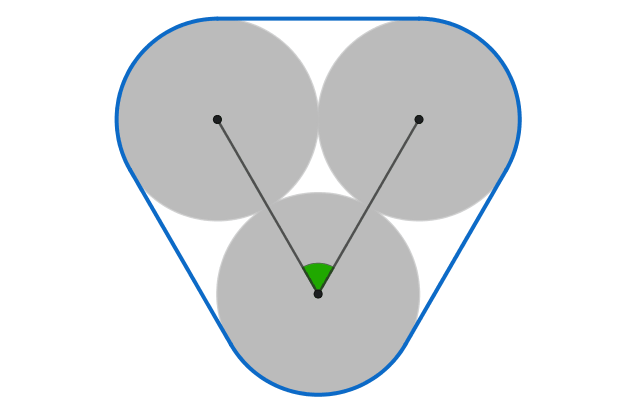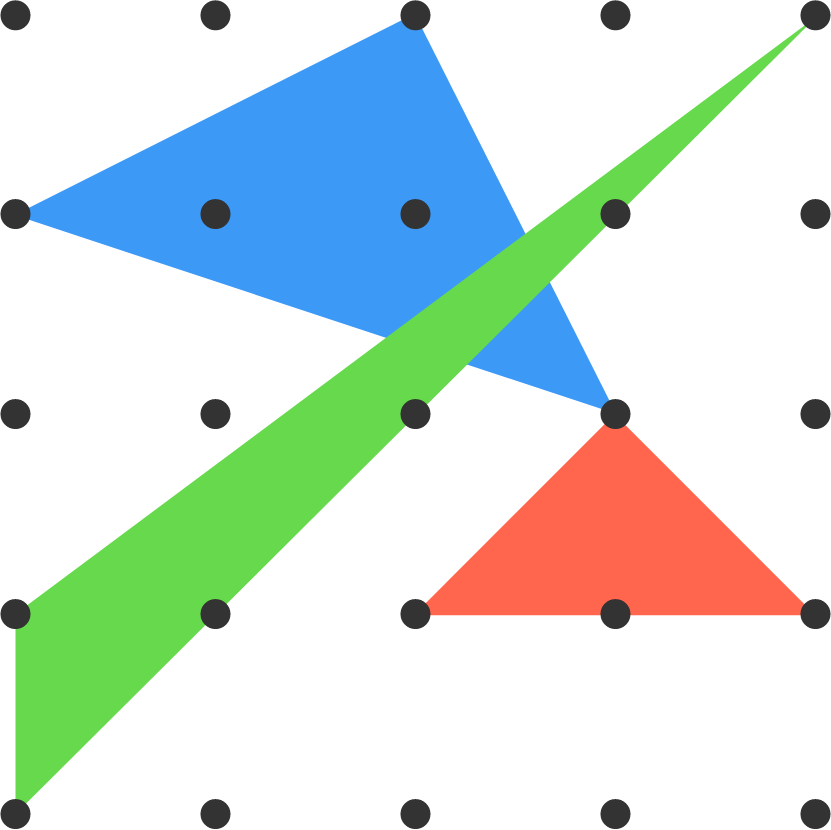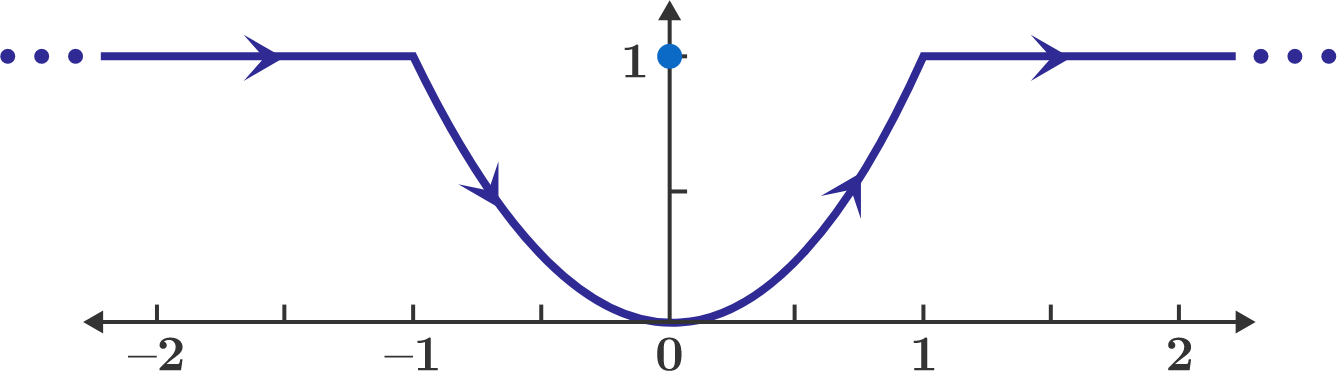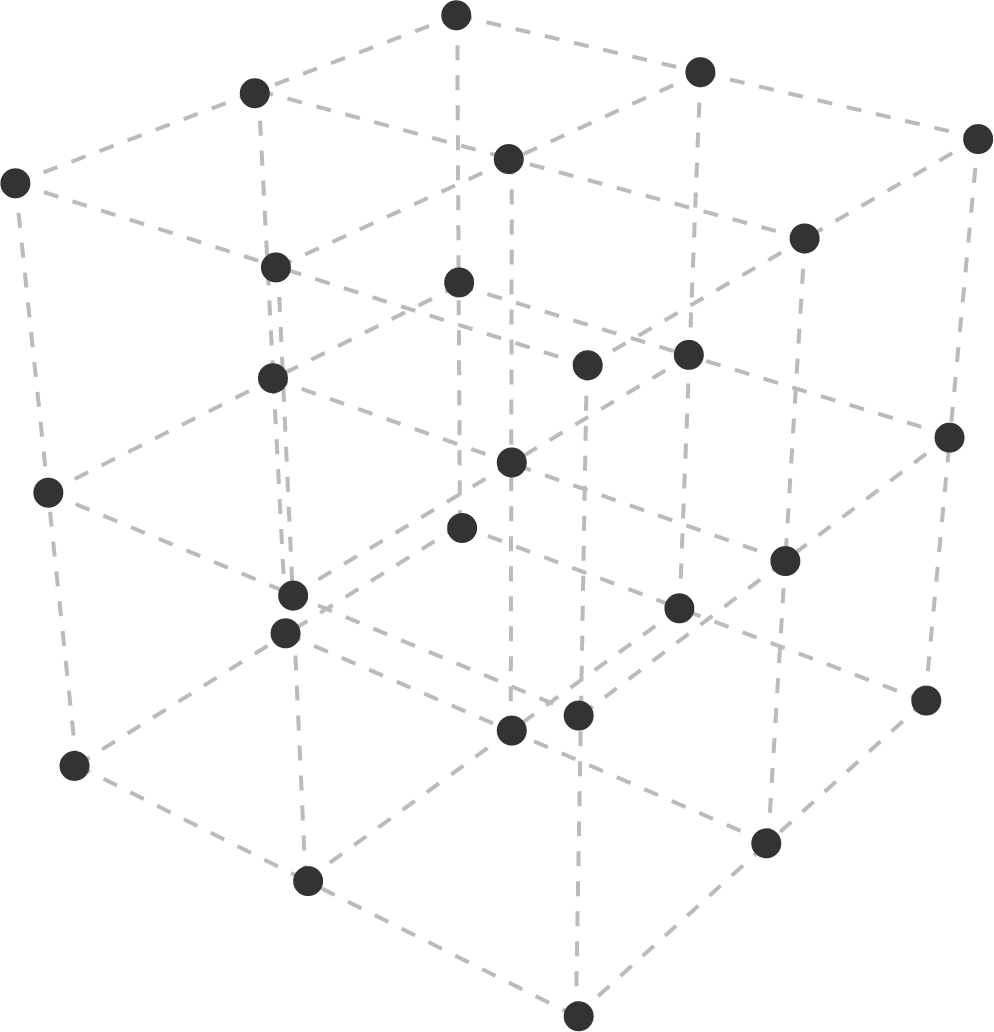# Problems of the Week

Contribute a problem

# 2018-05-07 AdvancedThe centers of three identical coins form the angle that's colored green above.

What angle maximizes the area of the blue convex hull?

Note: You can imagine the perimeter of the convex hull as a rubber band stretched around the three coins.

How many triangles can you make using points on this $5\times 5$ square grid as their vertices?

Note: Some of the triangles can be congruent, and they can overlap one another.Three possible triangles are shown.

$\large \sum_{n=1}^{\infty}\frac1{n2^n}=\, ?$

A wire in the shape of the curve $y = x^2$ carries a current $I.$ It also contains some horizontal, semi-infinite segments.

The magnitude of the magnetic flux density $(B)$ at the point $(x,y) = (0,1)$ is $B = \alpha \, \frac{\mu_0 \, I}{4 \pi}.$ If $\alpha$ is a positive real number, what is its value?The figure shows part of an infinitely large cube lattice.

What percentage of points are visible from a given point in the infinite lattice (to the nearest integer)?

Note: A point is not visible if it is directly behind another point in the line of sight.

×

Problem Loading...

Note Loading...

Set Loading...